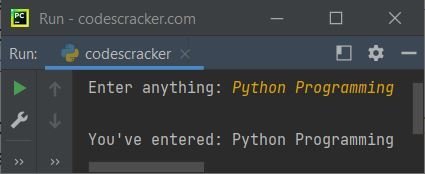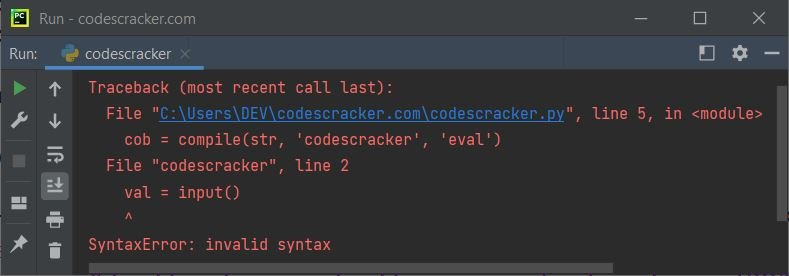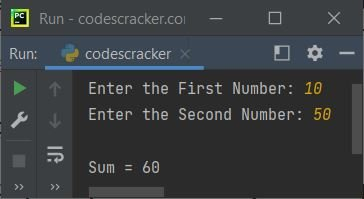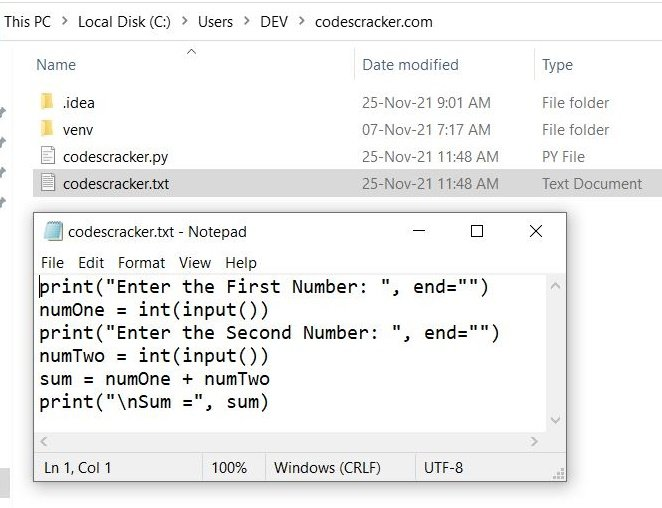# Python compile() Function

The compile() function in Python returns a code object of the specified source. This function is used when we need to compile a string as a code. For example:

```mycode = compile('print("python programming")', 'codescracker', 'eval')
exec(mycode)```

Note: Most of the time, professional Python developer uses compile() with exec() function in metaprogramming. An example of metaprogramming is given at last of this article.

Note: The exec() function executes dynamically created codes.

## Python compile() Function Syntax

The syntax of compile() function in Python is:

`compile(source, filename, mode, flags, dont_inherit, optimize)`

where:

• source - is a source that needs to be compile. A String, Bytes, or an AST (Abstract Syntax Tree) object is allowed as type of source
• filename - is a name of file from where the source code needs to be compiled. If you're not using an external file to compile the code from, then you can write whatever you want
• mode - is used tell the type of expression to execute. The value of mode can be one of the following three:
• eval - if the code available in source is a single expression
• single - if the code available in source is a single interactive statement
• exec - if the code available in source is a block of statements
• flags - is used to define the way to compile the source
• dont_inherit - is also used to define the way to compile the source. This parameter comes in boolean value. That is, either True or False, the one from these two values can be given to this parameter
• optimize - is used when we need to define the optimization level of the compiler

Note: The first three, that are source, filename, and mode parameters are required. Whereas all other parameters are optional.

Note: The default value of flags is 0, dont_inherit is False, and optimize is -1

## Python compile() Function Example

Because the syntax of compile() function is covered. Therefore it is the time to get some examples of this function to understand implementation.

### Use compile() Function to Compile a String as Code

Here is an example that compiles a string as a code, using of course, the compile() function in Python:

```str = "print(\"Enter anything: \", end=\"\")"
str = str + "\n" + "val = input()"
str = str + "\n" + "print(\"\\nYou've entered:\", val)"

cob = compile(str, 'codescracker', 'exec')

exec(cob)```

The snapshot given below shows the sample run of this Python program, with user input Python Programming:The above program can also be created in this way:

```str = "print(\"Enter anything: \", end=\"\")" + "\n" + "val = input()" + "\n" + "print(\"\\nYou've entered:\", val)"

cob = compile(str, 'codescracker', 'exec')

exec(cob)```

Since the first statement becomes too long, therefore I've split the statement in way that each line is used to add one statement inside the str, a String type variable.

I've used exec as mode, because str contains block of statements. But if you will not, that is, if you write any of the other two say eval as mode, then the compile() function raises an exception named SyntaxError, like shown in the snapshot given below:This is because, after using the eval as mode, meaning that the compile() function treats the source object containing a single expression. But it contains a block of statements. Therefore we're seeing this error. This error can be removed either by using the correct mode, or by catching the exception using the except block. The second way is actually not removing the error, rather it produces your manual error message. Here is the modified version of previous program:

```str = "print(\"Enter anything: \", end=\"\")"
str = str + "\n" + "val = input()"
str = str + "\n" + "print(\"\\nYou've entered:\", val)"

try:
cob = compile(str, 'codescracker', 'eval')
exec(cob)
except SyntaxError:
print("\nSomething went wrong!")```

Now the output would be:

`Something went wrong!`

### Indentation is Important while Putting the Code in Source Parameter

Here is another program that again uses a string variable str that contains a block of statements with some conditional statements. Then the variable is used as source for compile() function to execute the code available in the string.

```str = "print(\"Enter a Number: \", end=\"\")"
str = str + "\n" + "num = int(input())"
str = str + "\n" + "if num%2 == 0:"
str = str + "\n" + "    print(\"\\nEven Number\")"
str = str + "\n" + "else:"
str = str + "\n" + "    print(\"\\nOdd Number\")"

cob = compile(str, 'codescracker', 'exec')

exec(cob)```

This program basically checks whether a number entered by user is an even or an odd number. The sample run with user input 13 is shown in the snapshot given below:The interesting question that may arise in your mind is, what is the type of variable cob ?
Here is another program I've created to check it out. I know it is a code type object. But let's create an example to print the type using the program:

```cob = compile('print(100)', 'codescracker', 'eval')
print(type(cob))```

The output produced by this program, is:

`<class 'code'>`

### Metaprogramming using compile() in Python

The compile() function in Python is also used to do metaprogramming. In metaprogramming, we use a program as the data of another program. For example, the program given below uses the content of a file named codescracker.txt as code data for the program:

```fileObject = open("codescracker.txt", "r")
fileObject.close()

codeObject = compile(fileHandle, "codescracker.txt", "exec")
exec(codeObject)```

The sample run of this program, with user input 10 and 50 is shown in the snapshot given below:The file codescracker.txt with code must has to be available inside the current directory. Here is the snapshot of the current directory with opened file:This is just a simple program demonstrating how the compile() function can be used to execute code from an external file.

Note: The detailed description about open(), read(), and close() methods are given in its separate tutorials.

Python Online Test

« Previous Function Next Function »